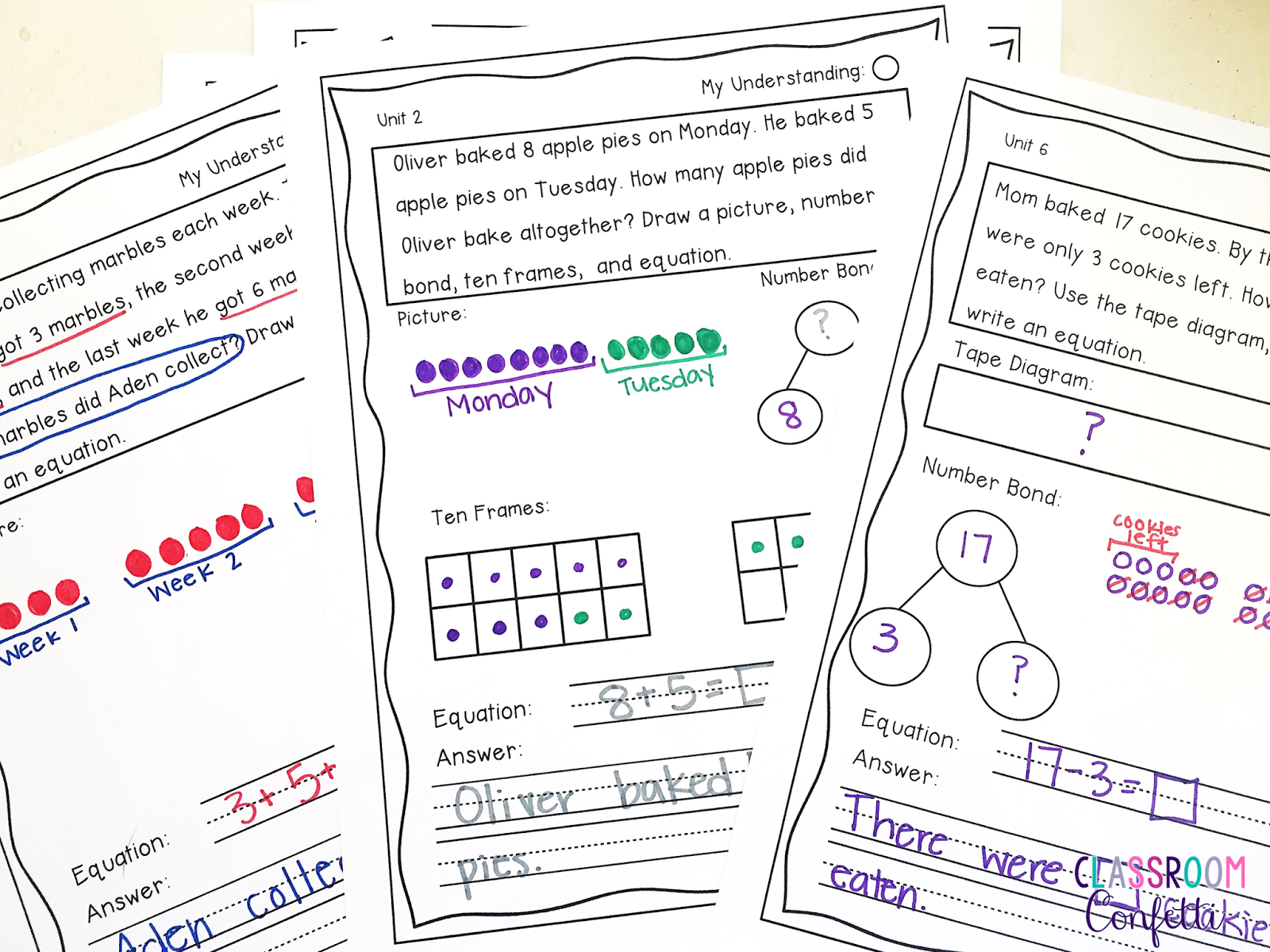### How To Use Problem Solving in Math Everyday

One of my favorite times of the school day is when my students and I begin our math problem solving. These 15 minutes are packed with not only mathematical skills and higher order thinking skills, but also with speaking and listening skills. Students love it, I love it, we are all happy.

I begin my math block with problem solving by using math journals that students keep at their desk or table. We all open up to the next problem and read it together. We underline key words, act out the problem, do a "Mind Movie," among other various strategies together. These beginning activities are done to get students thinking about the problem by using analytical skills. Through the year this is scaffolded down to being done independently or in small groups. We take about 2-3 minutes to get our brains ready to solve.

A key to underline or note is "What is the problem asking?" and "What do you already know?" These two ideas are crucial to learning to solve problems.

At this point students begin to solve the problem independently using strategies such as drawing picture, using a number line, using manipulatives, etc. Students almost always write an equation (when appropriate) and answer the question using a sentence. They usually work independently for about five minutes. Find First Grade Math Journals Here.

Students need to answer the question being asked by the problem.

At this point we begin to break down the problem together using some math talk. Two separate students are chosen to present their way of solving the problem. The presenting student is not only using math talk to explain their mathematical reasoning, but also practicing their speaking skills. It is important the class is listening because when the presenter is done two different students are chosen to ask the presenter a question about their problem solving.

Questions such as "How did you know that was your answer?" or "Why did you add instead of subtracting?" are great ideas to discuss as a whole class and bring up main teaching points.Find these First Grade Math Journals Here
After discussing the problem in depth through presenting and question & answer we finish our problem solving and move into our math lesson for the day. The process takes about 15 minutes and is simple to incorporate into your daily math program. Find First Grade Math Journals here.

You can find any of the math journals discussed in this post by clicking on any of the pictures or by clicking here.

Happy Problem Solving!

1.2.3.